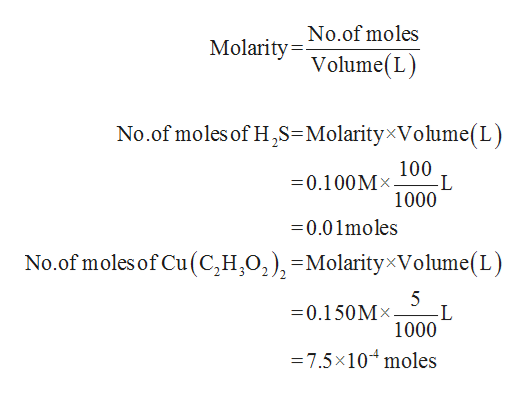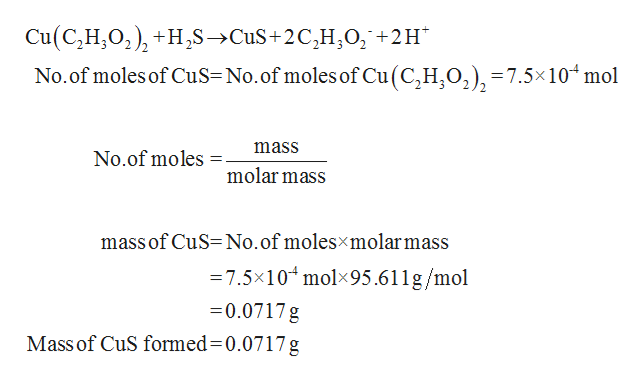# determine the mass of CuS formed when 100.ml of .100 M H2s and 5.00 ml of .150 M Cu(c2H3O2)2 are reacted. What is the concentration of C2H3O2- in the resulting solution?

Question
2 views

determine the mass of CuS formed when 100.ml of .100 M H2s and 5.00 ml of .150 M Cu(c2H3O2)2 are reacted. What is the concentration of C2H3O2- in the resulting solution?

check_circle

Step 1

Given the volume and molarity of H2S and Cu(C2H3O2)2. Using these, number of moles of both can be determined.help_outlineImage TranscriptioncloseMolarity No.of moles Volume(L) No.of moles of H,S=MolarityxVolume(L) 100 -L 1000 0.100Mx 0.01moles No.of moles of Cu (C,H,02), -MolarityxVolume(L) 5 L 1000 0.150Mx -7.5x104 moles fullscreen
Step 2

From the equation for the given reaction, it can be seen that one mole of Cu(C2H3O2)2 will give one mole of CuS. Therefore, the number of moles o...help_outlineImage TranscriptioncloseCu (C,H,02H,S>CuS+2CH0, +2H No.of moles of CuS- No.of moles of Cu (C,H,02), = 7.5x 104 mol mass No.of moles 1 molar mass massof CuS- No.of molesxmolarmass =7.5x104molx95.611g/mol 0.0717 g Mass of CuS formed=0.0717g fullscreen

### Want to see the full answer?

See Solution

#### Want to see this answer and more?

Solutions are written by subject experts who are available 24/7. Questions are typically answered within 1 hour.*

See Solution
*Response times may vary by subject and question.
Tagged in